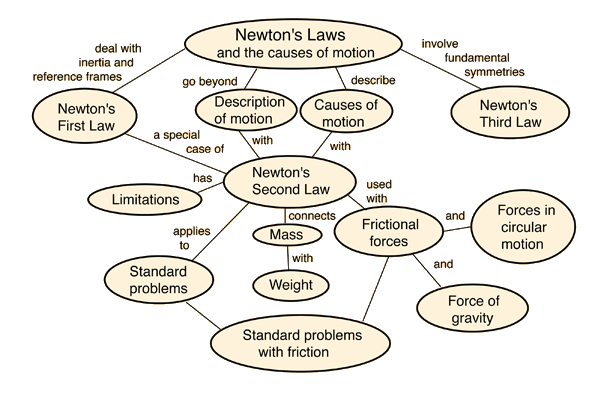Newton's 3rd law

# Syntax# Overview

Newton's third law stats that for every action there is an equal and opposite reaction.
The statement means that in every interaction, there is a pair of forces acting on the two interacting objects. The size of the forces on the first object equals the size of the force on the second object. The direction of the force on the first object is opposite to the direction of the force on the second object. Forces always come in pairs - equal and opposite action-reaction force pairs.

# Equations

## Equation One

-This supports LaTeX!

A 15-lb block rests on the floor.
(a) What force does the floor exert on the block?
(b) If a rope is tied to the block and run vertically over a pulley and the other end is attached to a free-hanging 10-lb weight, what is the force exerted by the floor on the 15-lb block?
(c) If we replace the 10-lb weight in part (b) with a 20-lb weight, what is the force exerted by the floor on the 15-lb block?

hile a football is in flight, what forces act on it? What are the action and reaction pairs while the football is being kicked and while it is in flight?

# Special Cases kind of

Newton's third law is one of the fundamental symmetry principles of the universe. Since we have no examples of it being violated in nature, it is a useful tool for analyzing situations with are somewhat counter-intuitive. For example, when a small truck collides head-on with a large truck, your intuition might tell you that the force on the small truck is larger. Not so!

# ApplicationsConsider the flying motion of birds. A bird flies by use of its wings. The wings of a bird push air downwards. Since forces result from mutual interactions, the air must also be pushing the bird upwards. The size of the force on the air equals the size of the force on the bird; the direction of the force on the air (downwards) is opposite the direction of the force on the bird (upwards). For every action, there is an equal (in size) and opposite (in direction) reaction. Action-reaction force pairs make it possible for birds to fly.